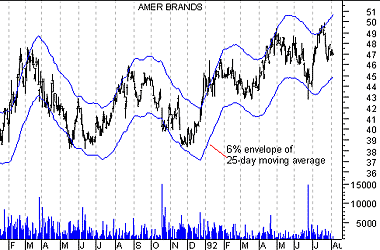Please enable JavaScript to view this page content properly Log In | Sign Up

Overview

An envelope is comprised of two moving averages. One moving average is shifted upward and the second moving average is shifted downward.

Interpretation

Envelopes define the upper and lower boundaries of a security's normal trading range. A sell signal is generated when the security reaches the upper band whereas a buy signal is generated at the lower band. The optimum percentage shift depends on the volatility of the security--the more volatile, the larger the percentage.

The logic behind envelopes is that overzealous buyers and sellers push the price to the extremes (i.e., the upper and lower bands), at which point the prices often stabilize by moving to more realistic levels. This is similar to the interpretation of Bollinger Bands.

Example

The following chart displays American Brands with a 6% envelope of a 25-day exponential moving average.You can see how American Brands' price tended to bounce off the bands rather than penetrate them.

Calculation

Envelopes are calculated by shifted moving averages. In the above example, one 25-day exponential moving average was shifted up 6% and another 25-day moving average was shifted down 6%.

 EMA Envelope Action Price Crossed Above Upper Bound of EMA Envelope(50,15)Price Crossed Above Lower Bound of EMA Envelope(50,15)Price Crossed Below Upper Bound of EMA Envelope(50,15)Price Crossed Below Lower Bound of EMA Envelope(50,15)Price Touched Above Upper Bound of EMA Envelope(50,15)Price Touched Above Lower Bound of EMA Envelope(50,15)Price Touched Below Upper Bound of EMA Envelope(50,15)Price Touched Below Lower Bound of EMA Envelope(50,15)Price Bounced Up From Upper Bound of EMA Envelope(50,15)Price Bounced Up From Lower Bound of EMA Envelope(50,15)Price Bounced Down From Upper Bound of EMA Envelope(50,15)Price Bounced Down From Lower Bound of EMA Envelope(50,15)Price Is Above Upper Bound of EMA Envelope(50,15)Price Is Above Lower Bound of EMA Envelope(50,15)Price Is Below Upper Bound of EMA Envelope(50,15)Price Is Below Lower Bound of EMA Envelope(50,15)